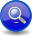#Boost C++ Libraries

...one of the most highly regarded and expertly designed C++ library projects in the world.

##### Expectation Operator (```a > b```)
###### Description

There are occasions in which it is expected that the input must match a particular parser or the input is invalid. Such cases generally arise after matching a portion of a grammar, such that the context is fully known. In such a situation, failure to match should result in an exception. For example, when parsing an e-mail address, after matching a name and "@" there must be a domain name or the address is invalid.

The expectation operator (>) requires that the following parser match the input or an exception is emitted. Using on_error(), that exception can be handled by calling a handler with the context at which the parsing failed can be reported.

By contrast, the follows operator (>>) does not require that the following parser match the input, which allows for backtracking or simply returning false from the parse() function with no exceptions.

Like the Sequence, the expectation operator, ```a > b```, parses two or more operands (`a`, `b`, ... etc.), in sequence:

```a > b > ...
```

However, while the plain Sequence simply returns a no-match (returns `false`) when one of the elements fail, the expectation: `>` operator throws an `expectation_failure``<Iter>` when the second or succeeding operands (all operands except the first) fail to match.

###### Header
```// forwards to <boost/spirit/home/qi/operator/expect.hpp>
#include <boost/spirit/include/qi_expect.hpp>
```

Also, see Include Structure.

###### Model of

Notation

`a`, `b`
`Iter`
###### Expectation Failure

When any operand, except the first, fail to match an `expectation_failure<Iter>` is thrown:

```template <typename Iter>
struct expectation_failure : std::runtime_error
{
Iter first;           // [first, last) iterator pointing
Iter last;            // to the error position in the input.
`info` what_;       // Information about the nature of the error.
};
```
###### Expression Semantics

Semantics of an expression is defined only where it differs from, or is not defined in `NaryParser`.

Expression

Semantics

```a > b```

Match `a` followed by `b`. If `a` fails, no-match. If `b` fails, throw an `expectation_failure<Iter>`

NoteIf you need an exception to be thrown if `a` fails, use the `expect` directive instead of the expectation operator.
###### Attributes

Expression

Attribute

```a > b```

```a: A, b: B --> (a > b): tuple<A, B>
a: A, b: Unused --> (a > b): A
a: Unused, b: B --> (a > b): B
a: Unused, b: Unused --> (a > b): Unused

a: A, b: A --> (a > b): vector<A>
a: vector<A>, b: A --> (a > b): vector<A>
a: A, b: vector<A> --> (a > b): vector<A>
a: vector<A>, b: vector<A> --> (a > b): vector<A>```

###### Complexity

The overall complexity of the expectation parser is defined by the sum of the complexities of its elements. The complexity of the expectation operator itself is O(N), where N is the number of elements in the sequence.

###### Example
NoteThe test harness for the example(s) below is presented in the Basics Examples section.

Some using declarations:

```using boost::spirit::ascii::char_;
using boost::spirit::qi::expectation_failure;
```

The code below uses an expectation operator to throw an `expectation_failure` with a deliberate parsing error when `"o"` is expected and `"i"` is what is found in the input. The `catch` block prints the information related to the error. Note: This is low level code that demonstrates the bare-metal. Typically, you use an Error Handler to deal with the error.

```try
{
test_parser("xi", char_('x') > char_('o')); // should throw an exception
}
catch (expectation_failure<char const*> const& x)
{
std::cout << "expected: "; print_info(x.what_);
std::cout << "got: \"" << std::string(x.first, x.last) << '"' << std::endl;
}
```

The code above will print:

```expected: tag: literal-char, value: o
got: "i"
```
 Copyright © 2001-2011 Joel de Guzman, Hartmut Kaiser Distributed under the Boost Software License, Version 1.0. (See accompanying file LICENSE_1_0.txt or copy at http://www.boost.org/LICENSE_1_0.txt)